Hm, so what's this you might ask. Well, nothing special :-) I decided to code some applets while I began to deal with Bezier Curves & the De Casteljau Algortihm to visualize some basics. I think they helped me to understand everything better so I decided to make them public. Note that even I write some text to some applets, these comments will probably not enough to understand what's really behind them. I'll try to give some good links but I cannot promise it.
This site will be slowly updated along I code new applets...

Note: The control of the applets should go without saying. The black points can be dragged with the left mousebutton. In some you can add new points with the left button and delete points by clicking them with the right mousebutton.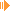Linear InterpolationCubic Bézier CurvesThe Bernstein Form of a Bézier CurveThe de Casteljau AlgorithmDegree Elevation

 Linear Interpolation (of two points)

The following applet is pretty simple: it visualizes the concept of linear interpolation of two points; let's call them a and b. The set of all points x of the form

 x = x(t) = (1-t)a + tb, t out of R

is called the straight line through a and b. For t = 0 the straight line passes through a and for t = 1 it passes through b. For 0 <= t <= 1 the point x is between a and b. x is represented as a barycentric combination of the two points a and b.

 Cubic Bézier Curves

The generalization of Linear interpolation leads us to Bézier curves. Say we have three points b0, b1 and b2. b1_0 and b1_1 are temporary points, b is the point on the curve. To obtain our point on the curve we do: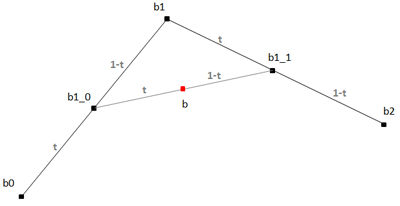b1_0 = (1-t)b0 + tb1 b1_1 = (1-t)b1 + tb2 b = (1-t)b1_0 + tb1_1

So from the two intermediate points b1_0 and b1_1 we calculate the final point on the curve b. Of course we can insert the first two equations in the third one to get a closed form:

 b = (1-t)b1_0 + tb1_1 = (1-t)((1-t)b0 + tb1) + t((1-t)b1 + tb2) = (1-t)²b0 + (1-t)tb1 + (1-t)tb1 + t²b2 = (1-t)²b0 + 2t(1-t)b1 + t²b2

This is a quadratic expression in t. Of course this could again be iterated to get the cubic curve with four so-called control points b0, b1, b2 and b3:

 b = (1-t)³b0 + 3t(1-t)²b1 + 3(1-t)t²b2 + t³b3

 The Bernstein Form of a Bézier Curve

To become more general, we can specify a Bézier curve in an explicit form. That's why we will describe Bézier Curves in terms of Bernstein polynomials, defined byThe following applet draws the Bernstein polynomials of chosen degree. Note that the polynomials form a partition of unity (they sum up to one for every t). Note also that each polynomial is for 0 < t < 1 not equal zero which means that every control point influences the whole curve!

The have also other properties, for example they satisfy the following recursion: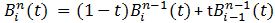Beside other properties (which I don't enumerate here) , the corresponding point on the curve is given by: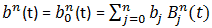The following applet shows a Bezier curve of arbitrary degree n which it is calculated by the explicit form:

 The de Casteljau Algorithm

One problem is that the evaluation with the Bernstein polynomial needs pretty much calculation power. De Casteljau invented an algorithm which calculates a point on the curve by recursive division of lines.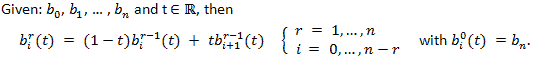is the point with parameter t on the bezier curve. That means you have to iterate n times to get a point on the curve. The polygon formed by b0, ... , bn is called the Bézier polygon or the control polygon of the curve. The polygon vertices bi are called control points or Bézier points.
You can also say that a Bézier curve of degree n can be defined by two Bézier curves of degree n-1.
The intermediate coefficients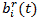can be written into a triangle array of points, the de Casteljau scheme. The following example is for the cubic case: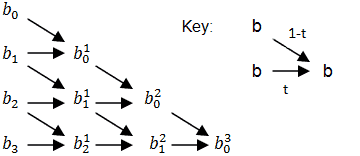The following applet implements this algorithm in a straight-forward recursive way to draw a bezier curve of arbitrary degree n by recursivly subdivide it into control polygons which can be approximated by a line after several steps (see ):

 Degree Elevation

Sometimes you have a Bézier curve of degree n. After modifying the curve you find out that you need one more control point. So you want to raise the degree by one to n+1 without changing the existing curve. Let's call the new control points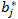. So following equation must be satisfied: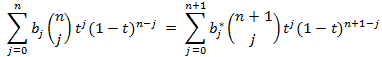("old curve = new curve")

Let's multiply the left side with t+(1-t) (which is one) and we get: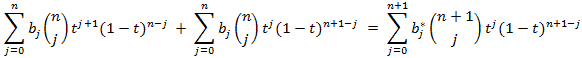Comparing the coefficients of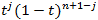on both side and we get: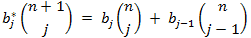or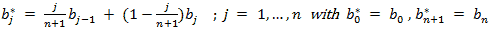So the new control points are calculated from the old one by piecewiese linear interpolation with values j/(n+1). Following applet illustrates that:

References:

 Wikipedia (German De Casteljau Algorithm site)
 Curves and Surfaces For Computer Aided Geometric Design - A Practical Guide (3rd Edition) by Gerald Farin

to be continued...

This site is part of Sunshine's Homepage